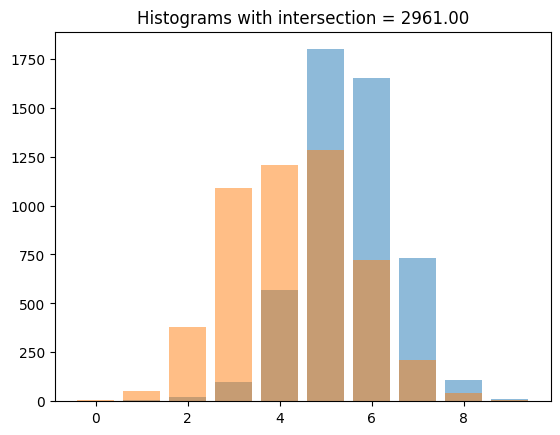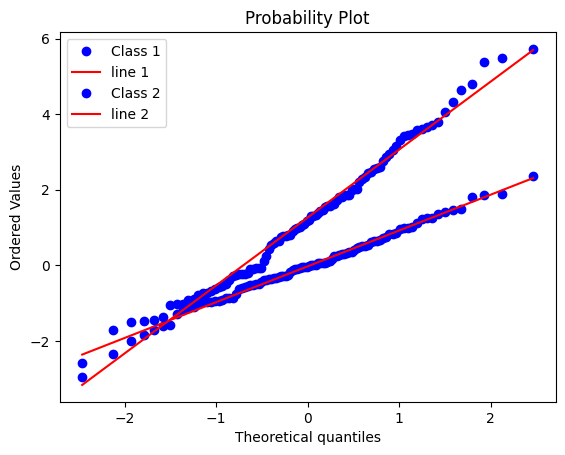# Histogram Intersection

Histogram intersection is a method for comparing two histograms, often used in image processing and computer vision. In machine learning, it can be used as a similarity metric for comparing features.

Here is an example of how histogram intersection can be used in a machine learning context, in a Jupyter notebook format:

In :
def histogram_intersection(hist1, hist2):
return np.sum(np.minimum(hist1, hist2))

In :
# Import necessary libraries
import numpy as np
import matplotlib.pyplot as plt
from sklearn.datasets import make_classification

# Generate data for histograms
X, y = make_classification(n_samples=1000, n_features=10, n_classes=2)
X_class1 = X[y == 0]
X_class2 = X[y == 1]

# Define histogram intersection function
def histogram_intersection(hist1, hist2):
return np.sum(np.minimum(hist1, hist2))

# Compute histograms for class 1 and class 2
hist1, _ = np.histogram(X_class1, bins=10)
hist2, _ = np.histogram(X_class2, bins=10)

# Compute histogram intersection
intersection = histogram_intersection(hist1, hist2)

# Plot histograms
plt.bar(range(10), hist1, alpha=0.5)
plt.bar(range(10), hist2, alpha=0.5)
plt.title("Histograms with intersection = {:.2f}".format(intersection))
plt.show()In this example, we use the make_classification function from sklearn to generate data for two classes. Then we use the np.histogram function to compute histograms for each class. Then we define a function for histogram intersection and apply it on the two histograms. Finally, we plot the histograms to visualize the result.

You can use the histogram intersection as a metric to compare features of different classes. For example, you can use histogram intersection as a similarity metric for comparing features of images in a dataset. The histogram intersection can also be used as a feature itself in a machine learning model, for example, as a part of a pipeline for image classification.

Please note that this is only one example of how histogram intersection can be used in machine learning, and there are many other ways it can be applied. Additionally, histogram intersection is one among many similarity metrics that can be used to compare histograms, and the choice of metric will depend on the specific use case.

The function histogram_intersection() takes in two histograms as input, represented as numpy arrays, and calculates the histogram intersection by summing the minimum values of each bin between the two histograms.

The numpy function np.minimum is used to compare the values of each bin between the two histograms and take the minimum value. Then the numpy function np.sum is used to sum the minimum values of all the bins, which gives the histogram intersection.

Please note that the above function assumes that the histograms have the same bin size and ranges. If the histograms have different bin size or ranges, you may need to normalize the histograms or bin values accordingly before applying the intersection.

Here is an example function that can be used to normalize two histograms to have the same bin size and range:

In :
def normalize_histograms(hist1, hist2, bins):
# Define bin edges for both histograms
bin_edges1 = np.linspace(np.min(hist1), np.max(hist1), bins + 1)
bin_edges2 = np.linspace(np.min(hist2), np.max(hist2), bins + 1)

# Compute histograms using the same bin edges
hist1_norm, _ = np.histogram(hist1, bins=bin_edges1)
hist2_norm, _ = np.histogram(hist2, bins=bin_edges2)

return hist1_norm, hist2_norm


This function takes in two histograms and the desired number of bins as input. First it computes the bin edges for both histograms using the np.linspace function. It takes the min and max value of the histograms as the range and the number of bins as an input. Then it computes the histograms using the same bin edges, using the np.histogram function.

You can use this function to normalize the histograms before applying the histogram intersection.

In :
hist1, hist2 = normalize_histograms(hist1, hist2, bins=10)
intersection = histogram_intersection(hist1, hist2)


## What are the other metrics that can be used to compare histograms.¶

There are many metrics that can be used to compare histograms, some of the most commonly used ones include:

• Intersection: As I have previously explained, it is a simple but widely used measure, which counts the number of bins where the histograms overlap. This metric gives a value between 0 and the minimum number of samples in the two histograms, with 0 indicating no overlap and the maximum value indicating perfect overlap.

• Chi-Squared Distance: The Chi-Squared distance is a measure of the dissimilarity between two histograms. It compares the observed histogram to a model histogram and calculates the sum of the squared differences between the observed and model histograms, normalized by the model histogram.

• Bhattacharyya Distance: The Bhattacharyya distance is a measure of similarity between two histograms. It is based on the Bhattacharyya coefficient, which is a measure of the similarity of two probability distributions.

• Earth Mover's Distance (EMD): The Earth Mover's Distance (EMD) is a measure of the difference between two histograms. It is based on the idea of how much "work" would be required to transform one histogram into the other.

• Kullback-Leibler divergence : Kullback-Leibler divergence is a measure of the difference between two probability distributions. It is commonly used for comparing histograms.

• Wasserstein Distance: Also known as the "Earth Mover's Distance" (EMD), it is a distance measure between probability distributions. It is widely used in image processing and computer vision, and has been applied to the comparison of histograms.

These are some examples of the widely used metrics to compare histograms, but there are many other methods that can be used. I recommend you to read more about them on this website: https://en.wikipedia.org/wiki/Histogram_comparison and consult further literature to find the best one for your use case.

## Q-Q plot¶

A two-group q-q plot, also known as a quantile-quantile plot, is a graphical method for comparing two histograms. It is used to assess whether two samples come from the same distribution by plotting the quantiles of one sample against the quantiles of the other sample.

The basic idea behind a two-group q-q plot is to compare the quantiles of the two histograms. The quantiles of a histogram are the points that divide the histogram into equal parts. The first quantile is the lowest point at which 25% of the data falls below, the second quantile is the point at which 50% of the data falls below, and so on.

To create a two-group q-q plot, you first need to compute the quantiles of each histogram. Then you plot the quantiles of one histogram on the x-axis and the quantiles of the other histogram on the y-axis.

If the two histograms come from the same distribution, the points on the plot should be approximately on a line with a slope of 1. If the two histograms come from different distributions, the points on the plot will deviate from a straight line, indicating that the distributions are different.

Here is an example of how to create a two-group q-q plot in Python:

In :
import numpy as np
import matplotlib.pyplot as plt
from scipy.stats import probplot

# Generate data for histograms
X_class1 = np.random.normal(loc=0, scale=1, size=100)
X_class2 = np.random.normal(loc=1, scale=2, size=100)

# Create two-group q-q plot
fig, ax = plt.subplots()
probplot(X_class1, plot=ax) # label='Class 1'
probplot(X_class2, plot=ax) # label='Class 2'
ax.legend(['Class 1','line 1','Class 2','line 2'])
plt.show()